Want to share your content on R-bloggers? click here if you have a blog, or here if you don't.

UPDATE: holy open source to the rescue. I posted a question yesterday on a idea peter had. Transaprency for overlaying light maps onto google maps. reminds me of the old Quake days. Well, I know John Carmack, John is an old friend of mine, but I’m no John Carmack. Neither am I Dr Paul Murrell. He whipped out some code, took my sample files and produced this beauty. Expect a post once I figure out how the hell he did that! light map, (blacker is brighter) dang i feel stupid.And you see that little patch of light at around 40.908, -4.1138

here’s what is there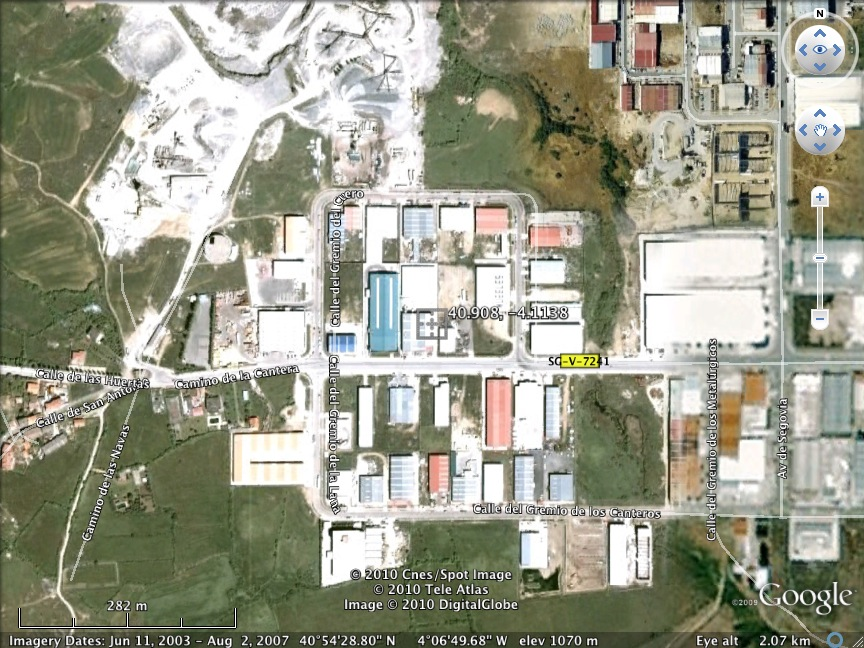Pretty good huh. Except, the station is in NAVACERRADA. which is just a little south of the “station location” Not far, just a couple decimal points away. In a peri urban/urban location that happens to be close to that 40 nightlights area, urban. Errors in station location matter. At least to the categorization…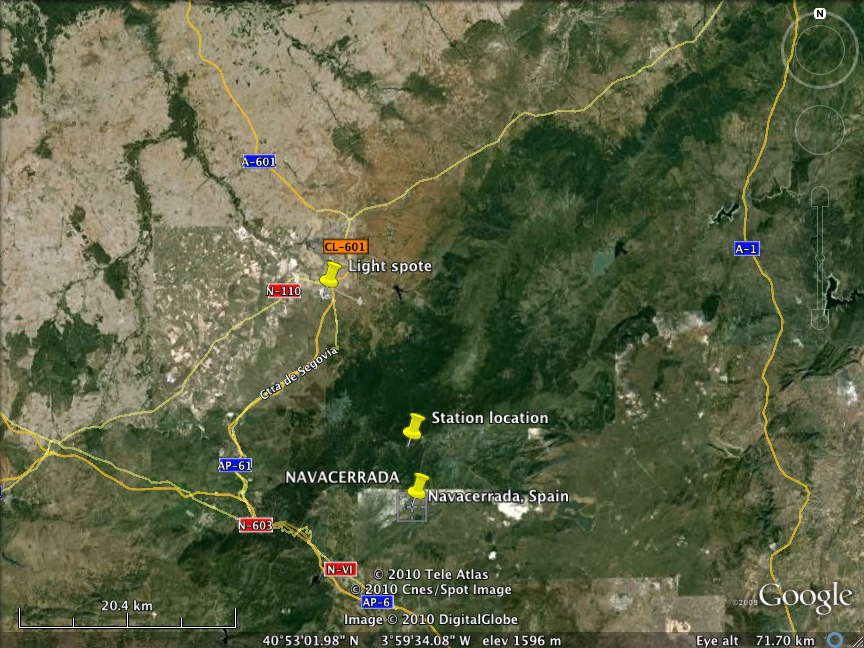A while back Ron Broberg and Peter O’Neill started down the path of understanding and replicating NASA’s use of nightlights. Ron’s latest effort is here. Peter’s work can be found here. My work has relied heavily on their insights and some of their trials and tribulations. I’ve had my own share of  great insights smashed upon looking at the code and data one last time and finally I’ve been able to replicate what GISS have done. This post will be long with a lot of code and charts. It assumes a familiarity with the Nightlights file and how NASA uses it.

In Ron’s last post he was trying to use “raster” to read in the “nightlights” file and he remarked that he could not match GISS results. Peter has also noted some irregularities. Ron notes that his results seemed shifted a cell in one direction or another. To me that seemed like a boundary problem so I spent time chasing down the boundary conditions ( when a station lands on a boundary) That was wrong. After a visitor noted some ‘registration’ problems and after Robert had a look at the file the source of the problem was clear: when the nightlights file was turned into a GeoTiff the metadata was not written correctly. the extents and pixel size were off by fractions. This does not impact GISS. Looking at the file they posted it was clear they were not using a *tif file, but rather a *.dat file prepared for them by Imhoff. I’ve written Imhoff to alert him to the issue with the *tif. In the meantime , with robert’s help I’ve hacked my way around the bad metadata and the results are a very close match with GISS.  To the code:

```source("Global.R")
dir<-myDirCreate("GissNightLights")
hiResLights<-raster(hiResNightlights)
extent(hiResLights) = c(-180,180,-90,90)
```

First we load the “world_ave.tif” into a raster. This is a 30 arcsecond file with nightlights every 1km. Recall these are derived from 2.7km pixels so the 1km resolution is really false. Next, we force an extent command onto the raster. Raster reads it extent from the file’s metadata. In this case the files metadata indicated the wrong extent. It left off a tiny sliver of the world. The resolution was consequently figured as .00833 degrees. That little imprecision was enough to cause big differences between GISS results and raster results. With that fixed, we proceed to load the Giss inventory. In previous programs I have modified this to hold information about the entire cell surrounding the station. The reason for that is the station location uncertainty. Next we will create some variables to categorize nightlights according to Imhoffs schema. We use ‘cut’ to recode the DN numbers into his categories, and we use “cut” to turn DN into GISS categories of “urban” “periurban” and “rural.” The we create a bar plot to compare NASA’s lights value from their inventory to our independent raster version. Finally we append the new variables to out inventory data frame.

```urbanType <- cut(Inv\$DSMP,c(-1,10,20,35,49,81,91,254,255),
labels=c("Rural","peri1","peri2","Urban1","Urban2","Urban3","Urban4","Saturated"))
NasaLights <-cut(Inv\$Nightlights,c(-1,10,35,255),right=T,
labels=c("Rural","Periurban","Urban"))
MoshLights <-cut(Inv\$DSMP,c(-1,10,35,255),right=T,labels=c("Rural","Periurban","Urban"))
Inv<-cbind(Inv,MoshLights,NasaLights,UrbanCode=urbanType)
h<-rbind(table(NasaLights),table(MoshLights))
fname <-fullPath(dir,"Stationtypes.png")
png(fname)
barplot(h,beside=T,legend.text=c("Giss","Raster"),col="blue")
dev.off()
```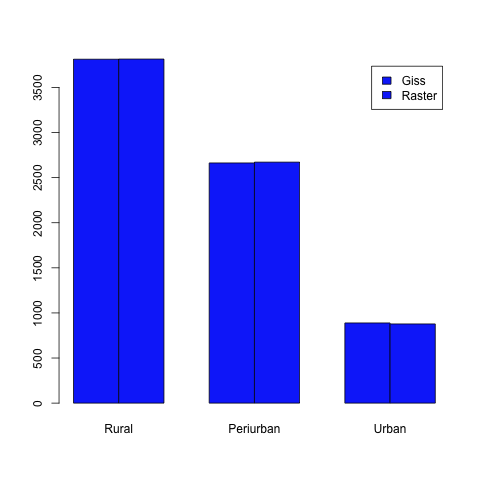As the chart indicates there is a very small difference between NASA’s categorization and our categorization. Still, there is a small difference, a handful of stations. And so we investigate the ‘spread’ between NASA nightlight values and the one we generate:

```

png(fname)
dev.off()
```The differences are minor and range from -67 to 31. This indicates that when each program does its lookup there are still some areas where we look up adjacent cells, but that difference does not result in changes to categorization. A brief check of the extrema in this case. First we look at the biggest positive difference and then the biggest negative difference. On second though I should probably turn this into an absolute difference.

```spread <- Inv\$Nightlights-Inv\$DSMP
e <-extent(c(Inv\$LonMin[dex],Inv\$LonMax[dex],Inv\$LatMin[dex],Inv\$LatMax[dex]))
r <-crop(hiResLights,e)
lightsPlot(r,Inv[dex,],dir)
print(Inv\$Id[dex])
```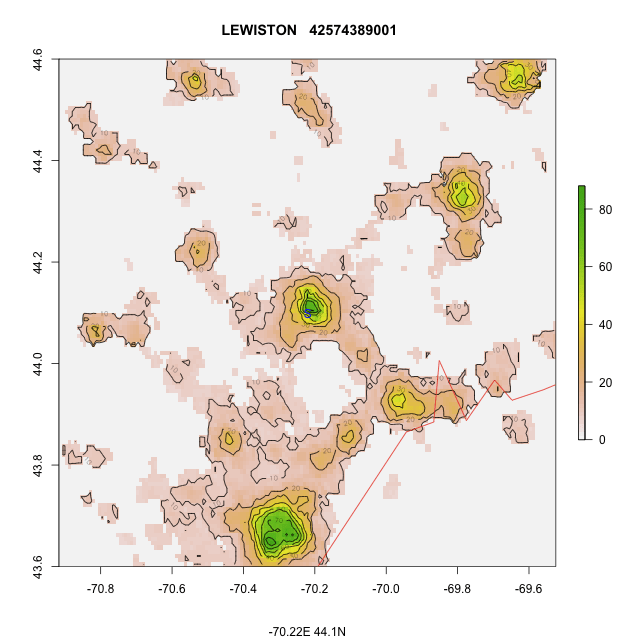Looking directly in the center you can see the ‘S’. And now for the biggest negative difference

```dex <- which(spread==min(spread,na.rm=T))
e <-extent(c(Inv\$LonMin[dex],Inv\$LonMax[dex],Inv\$LatMin[dex],Inv\$LatMax[dex]))
r <-crop(hiResLights,e)
lightsPlot(r,Inv[dex,],dir)
print(Inv\$Id[dex])
```This is harder to make out but at the center of this city we have, apparently, two cells close together that differ by 67. Lets look at the entire inventory for that location

Id:  22223074000 ;  Name: DUDINKA ;  Lat  69.4 ;Lon 86.17 ; Altitude 19 ; GridEl 50

Rural S ;  Population 20 (20,000)

Topography FL  ;Vegetation NA ; Coastal: no ; DistanceToCoast NA ; Airport  FALSE ;  DistanceToTown  NA

NDVI: WOODED TUNDRA

Light_Code C ( this is the deprecated GHCN light code)

US_LightCode NA ( this is the Giss 1,2,3 code: deprecated )

Nightlights  65 ( this is the value NASA sees when they look up the station location)

CountryIndex  86  CountryName   RUSSIAN FEDERATION (ASIAN SECTOR)   Cell 106779141 ( the cell id in raster)

DSMP  153 ( the value raster sees )

LonMin  84.75; LonMax 87.58333 ;LatMin 68.9; LatMax 69.9  ( the extent of the contour plot)

MinLights  0 ;MaxLights 166  ;MeanLights 0.7348775;  AreaLights 12353.27 (sqkm); SumLights 29983

the minimum, max, and mean lights within the extent. also the area of the extent and sum of all lights

PeriUrban 3km  Urban 3km:  how close  is the nearest periurban and urban light

MoshLights:Urban  NasaLights: Urban  UrbanCode: Urban4

The codes for urbanity, including the more detailed Imhoff code

PopDensity 3031.  the population density implied by the nightlights 3031 people sq/kmAnd wikipedia

Which indicates that the population figure in the  GISS inventory is not accurate. Next we look at the frequency of different types of lights we get at the stations:

```fname <-fullPath(dir,"DSMPHist.jpg")
png(fname,width=1024,height=480)
hist(Inv\$DSMP,breaks=25,main="Frequency of Nightlights at Station location", xlab="Radiance number",col="blue",xlim=c(0,max(Inv\$DSMP,na.rm=T)),ylim=c(0,5000))
dev.off()
```And then we will look through all the stations and create two categories. Stations where the surrounding area ( a 55km radius) is “rural” and those cells that have Periurban or urban lights in the vincinity

```AllRural <- ifelse(Inv\$MaxLights<11,T,F)
fname <-fullPath(dir,"RuralCells.jpg")
png(fname)
barplot(height=c(sum(AllRural==T),sum(AllRural==F)),main="Frequency of stations in Rural Cells",names.arg=c("Rural Cells","Mixed Cells"),col="blue")
dev.off()
```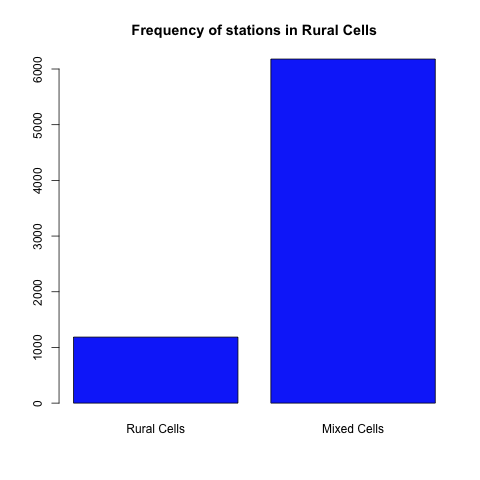And  then we select rural stations that have some peri/urban lights in the vicinity and we see how bight those nearby Lights are

```MixedCells <-Inv[which(Inv\$DSMP < 11),]
MixedCells <- MixedCells[which(MixedCells\$MaxLights > 10),]
fname <-fullPath(dir,"MixedCells.jpg")
png(fname,width=1024,height=480)
hist(MixedCells\$MaxLights,breaks=25,main="Frequency of Maxlights in Cell of Dark Stations", xlab="Radiance number",col="blue",xlim=c(0,max(MixedCells\$MaxLights,na.rm=T)),ylim=c(0,1000),labels=TRUE)
dev.off()
```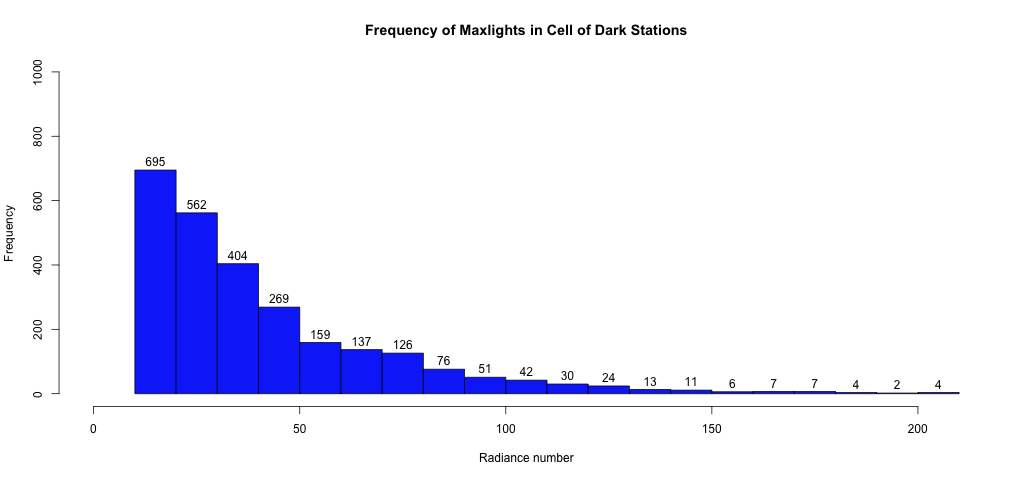Then we pull out the following. We look for the rural station that has the brightest nighlight within a 55km radius. And we plot that stations lightmap. I also write the small raster to disk. This is for the next project which is to test putting overlays onto google map! ( R list is helping so they need code and data!)And the code for that is below

```StationNumber <- which(MixedCells\$MaxLights==max(MixedCells\$MaxLights,na.rm=T))

e <-extent(c(MixedCells\$LonMin[StationNumber],
MixedCells\$LonMax[StationNumber],
MixedCells\$LatMin[StationNumber],MixedCells\$LatMax[StationNumber]))

r <-crop(hiResLights,e)
lightsPlot(r,MixedCells[StationNumber,],dir)
print(MixedCells\$Id[StationNumber])
fname <-fullPath(dir,"test.tif")
writeRaster(r,filename=fname,format="GTiff",datatype="INT1U",overwrite=T)
fname <-fullPath(dir,"test.grd")
writeRaster(r,filename=fname,format="raster",datatype="INT1U",overwrite=T)
```

Next we will select the Nasa stations that qualify under GISS criteria for Rural. And here we will look at the distance for the closest Periurban pixel. That is, the station has a value of 01-10. And we ask the question  How many rural stations have a peri urban pixel within 3km, within 5km, within 10km, 20km, 30km, 40km. Essentially if the station location is in error, even by a little, will changing the location put the station in a peri urban place. In short, I searched around every station and looked for bright pixels.

```NasaRural <- Inv[which(Inv\$NasaLights=="Rural"),]

fname <-fullPath(dir,"PeriUrbanDist.jpg")

png(fname,width=1024,height=480)

hist(NasaRural\$PeriUrban,main="Distance to Closest PeriUrban",col="blue",labels=TRUE,breaks=15,xlab="Distance(km)",xlim=c(0,50))

dev.off()
```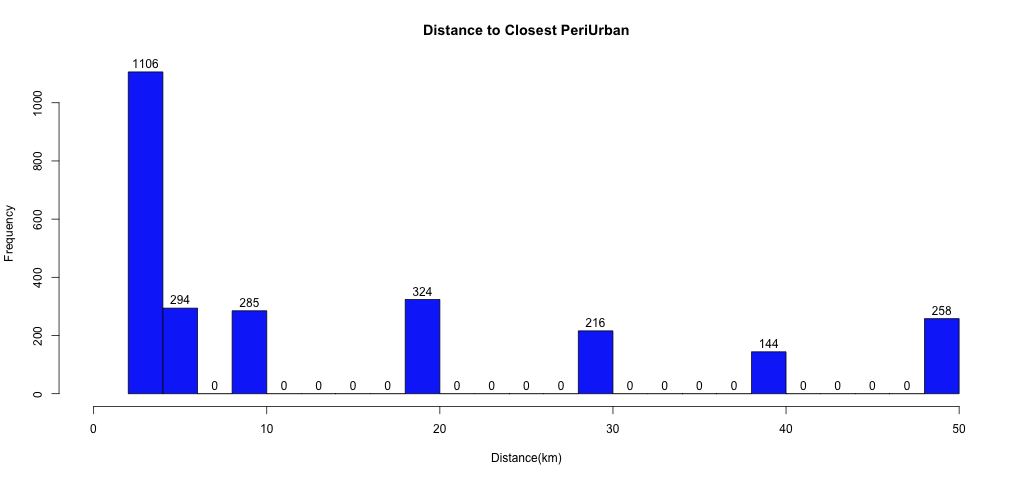Simply, if you look at Rural stations (around 3800)  1100 of those stations will have a peri urban pixel within 3km. hence, the accuracy of the station lat and lon will matter. 1400 of the stations have periurban within 5km and half are with 5km of a peri urban pixel. 5km is roughly .05 degrees. hence, accuracy in lat/lon matters. And in our survey we found stations that were mislocated by more than a full degree. Next, we look for nearby Urban pixels

```fname <-fullPath(dir,"UrbanDist.jpg")
png(fname,width=1024,height=480)
hist(NasaRural\$Urban,main="Distance to Closest Urban",col="blue",labels=TRUE,breaks=15,xlab="Distance(km)",xlim=c(0,50))
dev.off()
```Again, we take the rural stations and count how many stations have an urban pixel within 3km. That would be 10. How many have an urban pixel within 5km? 41, within 10km? 154. Location accuracy matters. Next, I noted that NASA have added a test looking at “pitch black” stations. That is, stations with light =0. What do these look like. First a count

```PitchDark <- ifelse(Inv\$DSMP==0,T,F)
fname <-fullPath(dir,"Pitchdark.jpg")
png(fname)
barplot(height=c(sum(PitchDark==T),sum(PitchDark==F)),
main="Frequency of Pitch dark stations in Giss Inv",
names.arg=c("Pitch dark Stations","Some Light "),col="blue")dev.off()
```The issue, however, is not with the actual value at the station (0-10) the issue is station location accuracy. What is needed is accurate location data. Sorting for pitch dark does no good if the location is wrong. To understand that we will start to look at the pixel surrounding a pitch black station.  Because of the location errors we start by screening at the CELL. Here we know this: we know that for these stations there are no lit pixels within 55km. So, inthese cases location error is not a factor. The station could be anywhere in this 1 degree cell and it would still be pitch dark. This is a conservative screen which would minimize misidentification of peri urban or urban sites as rural. Again, better location data and this screen becaomes less stringent

```DarkCells <- ifelse(Inv\$MaxLights==0,T,F)
fname <-fullPath(dir,"Darkcells.jpg")
png(fname)
barplot(height=c(sum(DarkCells==T),sum(DarkCells==F)),
main="Frequency of Pitch dark cells in Giss Inv",names.arg=c("Pitch dark Cells","Some Light "),col="blue")

dev.off()
```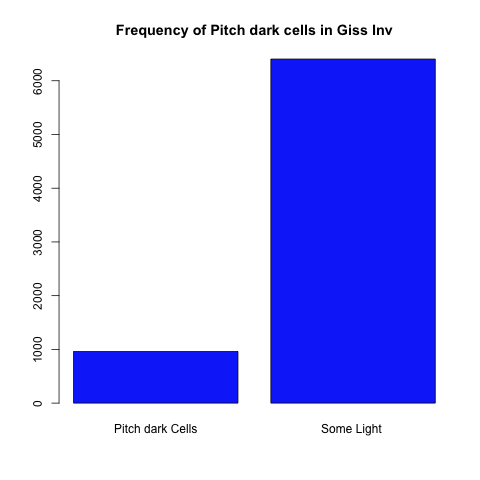So, there are roughly 1000 stations where the station is pitch dark and all the surrounding area ( 55km) is dark. And next we look at “dim” cells. This approach counts the stations that are in areas where the entire cell has DN numbers less than 11. All rural cells:

```DimCells <- ifelse(Inv\$MaxLights<11,T,F)
fname <-fullPath(dir,"Dimcells.jpg")
png(fname)
barplot(height=c(sum(DimCells==T),sum(DimCells==F)),
main="Frequency of Dim cells in Giss Inv",names.arg=c("Dim Cells","Some Light "),col="blue")
dev.off()
```As one might expect the number increases a bit. There are roughly 1200 stations where the  station and the surrounding area ( radius 55km) are all rural by Imhoff’s designation. So location error doesnt play here as well. Next we look, at pitch dark stations. And here we asses the pixels in the vicinity

```
fname <-fullPath(dir,"PeriPitchdark.jpg")
png(fname,width=1024,height=480)
hist(Inv\$PeriUrban[PitchDark],col="blue",labels=TRUE,breaks=20,xlab="Distance(km)",main="Occurance of peri urban by pitch dark stations",xlim=c(0,50))

dev.off()
```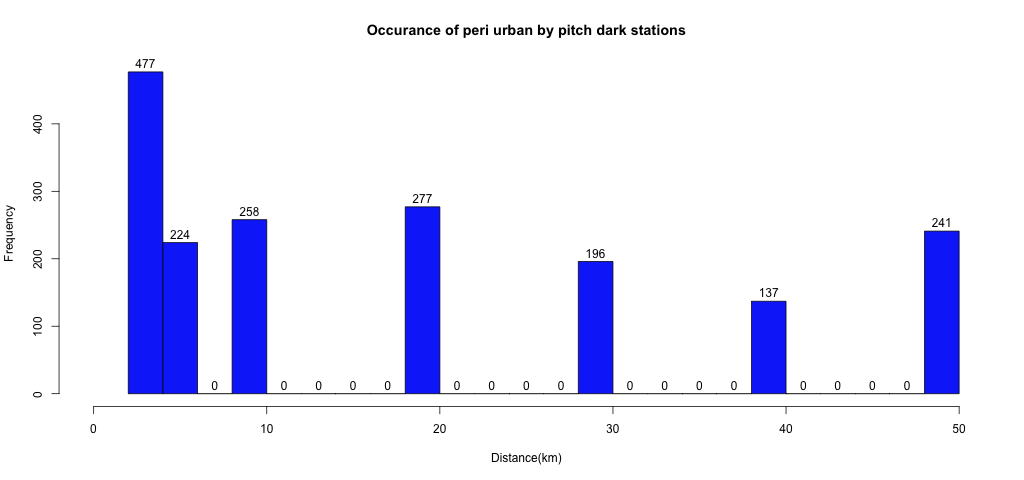The appraoch here is to look at pitch dark stations and then see what is within 3km, 5km etc.  Of all the pitch dark stations that NASA could select in its pitch dark test, 477 of them had periurban pixels within 3km, 224 stations had peri urban pixels within 5km. if the station location is off, then these stations would not be pitch dark.

Next we look at the occurance of urban pixels within these boundaries

```fname <-fullPath(dir,"UrbanPitchdark.jpg")
png(fname,width=1024,height=480)
hist(Inv\$Urban[PitchDark],col="blue",labels=TRUE,breaks=20,xlab="Distance(km)",main="Occurance of Urban by pitch dark stations",xlim=c(0,50))
dev.off()
```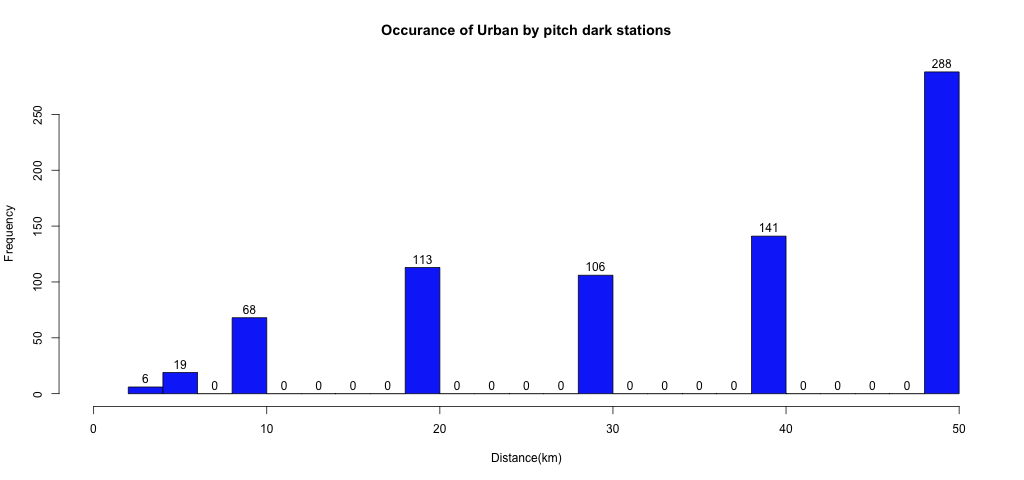So, if you look at all pitch dark stations, 6 of them have an urban pixel within 3km, 19 have an urban pixel within 5km. 68 have an urban pixel within 10km, 113 within 20km. and so forth. Finally, we look at 3 different kinds of sites. we count “pitch black cells, pitch black stations with rural lights for 55km, and finally rural cells. We’ve done these before but here we just put them on one chart

```siteType <- vector(length=3)
pdInv <- Inv[PitchDark,]
siteType <-nrow(pdInv[which(pdInv\$MaxLights == 0),])
siteType <-nrow(pdInv[which(pdInv\$MaxLights <  11),])
siteType <-nrow(Inv[which(Inv\$MaxLights <  11 ),])
fname <-fullPath(dir,"RuralcellType.jpg")
png(fname,width=1024,height=480)
barplot(height=siteType,main="1deg cell types",col="blue",names.arg=c("Black Cells","Dark Station/rural cell","Rural Cells"))
dev.off()
```Any of these screens would diminish the location  error problems. Finally, I take the data from Imhoff and I contstruct a field for population density in sq km. This gets added to the inventory. Theres more work to do here. Documenting pitch black stations with urban pixels nearby, fusion tables, google tours, overlays on google earth. Fun times ahead

```PopDensity <- vector(length=nrow(Inv))

for(i in 1:nrow(Inv)){

if(Inv\$UrbanCode[i]=="Rural")PopDensity[i]<-0.14

if(Inv\$UrbanCode[i]=="peri1")PopDensity[i]<-0.82

if(Inv\$UrbanCode[i]=="peri2")PopDensity[i]<-2.89

if(Inv\$UrbanCode[i]=="Urban1")PopDensity[i]<-6.32

if(Inv\$UrbanCode[i]=="Urban2")PopDensity[i]<-10.59

if(Inv\$UrbanCode[i]=="Urban3")PopDensity[i]<-18.66

if(Inv\$UrbanCode[i]=="Urban4")PopDensity[i]<-30.31

if(Inv\$UrbanCode[i]=="Saturated")PopDensity[i]<-NA

}

PopDensity <- PopDensity*100

Inv<-cbind(Inv,PopDensity)

fname <-fullPath(dir,"GissInvEnhanced.inv")

write.table(Inv,file=fname,row.names=F)
```